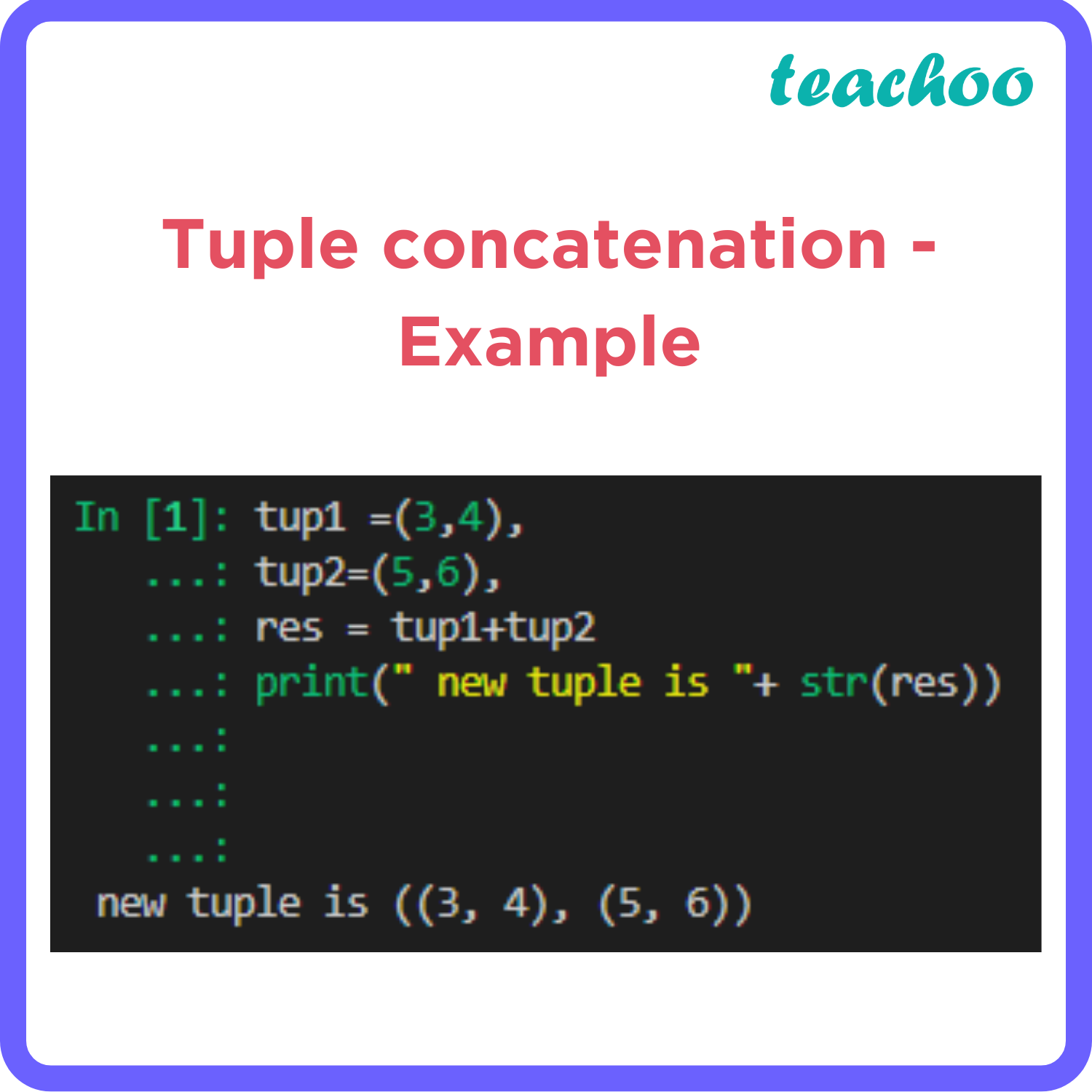Very Short Answer Type Questions (1 Mark each)

Computer Science - Class 11
Chapter 10 Class 11 - Tuples and Dictionaries

## ``` tup1 =(3,4), `````` tup2=(5,6), `````` res = tup1+tup2 `````` print(“ new tuple is "+ str(res)) ```The output of the code is:  new tuple is ((3, 4), (5, 6))

Explanation:

• The code creates two tuples, tup1 and tup2 , with two elements each.
• The code then uses the + operator to concatenate (join) the two tuples and assign the result to a new variable res.
•
• The + operator creates a new tuple that contains all the elements of the first tuple followed by all the elements of the second tuple.
• The code then prints the value of res using the str() function to convert it to a string and concatenating it with another string "new tuple is ".
• The print() function displays the output to the standard output device (usually the screen).

Learn in your speed, with individual attention - Teachoo Maths 1-on-1 Class